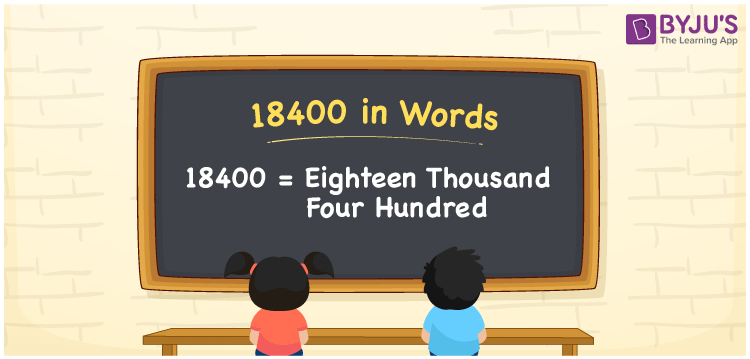# 18400 in Words

The number 18400 in words is “Eighteen Thousand Four Hundred”. The number 18400 is a natural number, and it can be expressed in words using the place value of a number. For example, Akshay bought a mobile for Rs. 18400. This can be written as “Akshay bought a mobile for Rs. Eighteen Thousand Four Hundred. In this article, we will discuss the spelling of 18400 and its procedure in detail.

 18400 in Words: Eighteen Thousand Four Hundred. Eighteen Thousand Four Hundred in Numerical Form: 18400.

## 18400 in English Words## How to Write 18400 in Words?

To convert 18400 in words, identify the place value of each digit of the given number. The following is the place value table for the number 18400.

 Ten-thousands Thousands Hundreds Tens Ones 1 8 4 0 0

Here is the expanded form for the number 18400:

= 1 × Ten thousand + 8 × Thousand + 4 × Hundred + 0 × Ten + 0 × One

= 1× 10000 + 8 × 1000 + 4 × 100

= 10000 + 8000 + 400

= 18400

= eighteen thousand four hundred

Hence, 18400 in words is Eighteen thousand four hundred.

18400 in words – Eighteen thousand four hundred

Is 18400 an odd number? – No

Is 18400 an even number? – Yes

Is 18400 a perfect square number? – No

Is 18400 a perfect cube number? – No

Is 18400 a prime number? – No

Is 18400 a composite number? – Yes

## Frequently Asked Questions on 18400 in Words

Q1

### Write 18400 in words.

18400 in words is eighteen thousand four hundred.

Q2

### Simplify 18000 + 400, and express in words.

Simplifying 18000 + 400, we get 18400. Hence, 18400 in words is Eighteen thousand four hundred.

Q3

### Convert eighteen thousand four hundred into numbers.

Eighteen thousand four hundred in numbers is 18400.